# Mathematics Support

### Transformations and Geometric Measurement

Congruence Through Rigid Transformations

Similarity Through Non-Rigid Transformations

Volumes of Cones, Cylinders, and Spheres

## Congruence Through Rigid Transformations

### Students will be able to...

• Verify experimentally the properties of rotations, reflections, and translations. Lines taken to lines (1a), line segments to line segments of the same length (1b); angles taken to angles of the same measure; parallel lines taken to parallel lines (1c).
• Understand that a two-dimensional figure is congruent to another if the second can be obtained from the first by a sequence of rotations, reflections, and translations; given two congruent figures, describe a sequence that exhibits the congruence between them.
• Describe the effect of dilations, translations, rotations, and reflections on two-dimensional figures using coordinates.
• Use informal arguments to establish facts about the angle sum and exterior angle of triangles, about the angles created when parallel lines are cut by a transversal, and the angle-angle criterion for similarity of triangles.

*Instructional videos in the hyperlinks above are meant to support C2.0 content, but may use vocabulary or strategies not emphasized by MCPS.

The Common Core State Standards require a balance of three fundamental components that result in rigorous mathematics acquisition: deep conceptual understanding, procedural skill, and mathematical applications and modeling.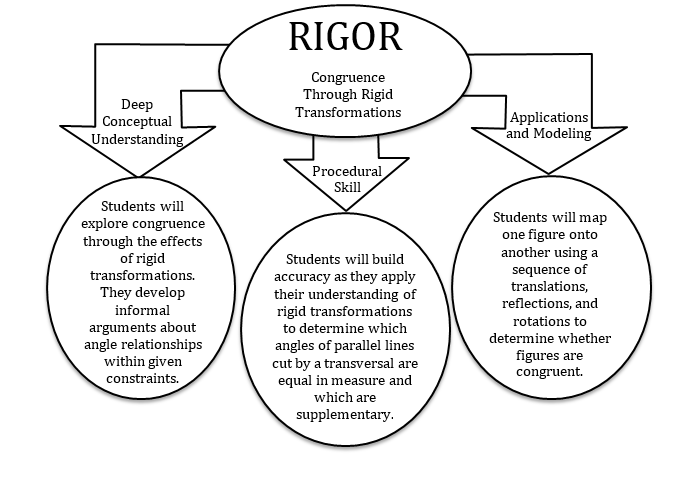### In school, your child will…

• Verify experimentally the properties of rotations, reflections, and translations. Lines taken to lines (1a), line segments to line segments of the same length (1b); angles taken to angles of the same measure; parallel lines taken to parallel lines (1c).
• Visit the online resource,  NLVM: Playing with Reflections
• Students click, drag, and rotate the shape on the right as they investigate the properties of rigid transformations.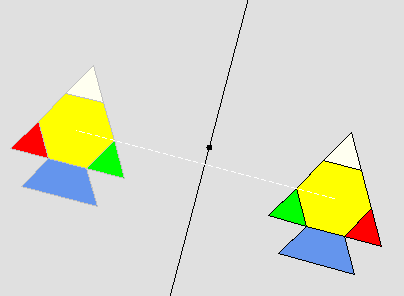• Understand that a two-dimensional figure is congruent to another if the second can be obtained from the first by a sequence of rotations, reflections, and translations; given two congruent figures, describe a sequence that exhibits the congruence between them.
• Describe the effect of dilations, translations, rotations, and reflections on two-dimensional figures using coordinates.
• Describe the sequence of transformations that moves the red triangle to triangle D.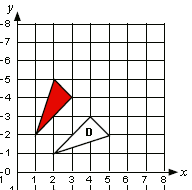• Use informal arguments to establish facts about the angle sum and exterior angle of triangles, about the angles created when parallel lines are cut by a transversal, and the angle-angle criterion for similarity of triangles.
• Find the measure of angle x. Present an informal argument showing that your answer is correct.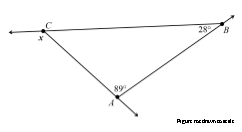### At home, your child can…

*Additional Practice links support C2.0 content, but may use vocabulary or strategies not emphasized by MCPS.

## Similarity Through Non-Rigid Transformations

### Students will be able to...

• Describe the effect of dilations, translations, rotations, and reflections on two-dimensional figures using coordinates.
• Understand that a two-dimensional figure is similar to another if the second can be obtained from the first by a sequence of rotations, reflections, translations, and dilations; given two similar two-dimensional figures, describe a sequence that exhibits the similarity between them.
• Use informal arguments to establish facts about the angle sum and exterior angle of triangles, about the angles created when parallel lines are cut by a transversal, and the angle-angle criterion for similarity of triangles. For example, arrange three copies of the same triangle so that the sum of the three angles appears to form a line, and give an argument in terms of transversals why this is so.

*Instructional videos in the hyperlinks above are meant to support C2.0 content, but may use vocabulary or strategies not emphasized by MCPS.

The Common Core State Standards require a balance of three fundamental components that result in rigorous mathematics acquisition: deep conceptual understanding, procedural skill, and mathematical applications and modeling.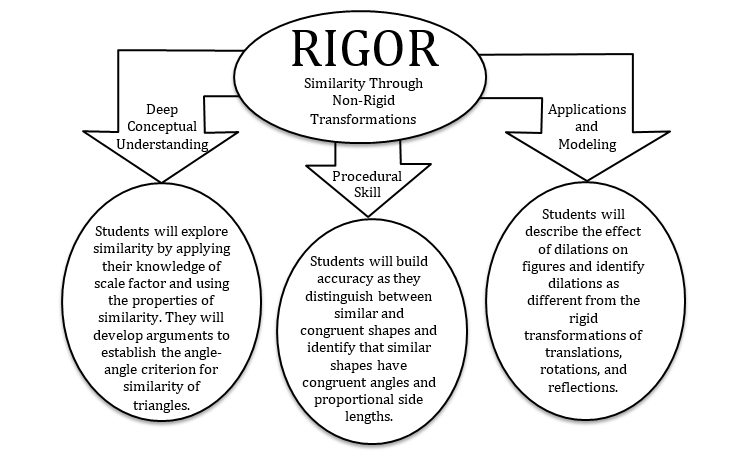### In school, your child will…

• Describe the effect of dilations, translations, rotations, and reflections on two-dimensional figures using coordinates.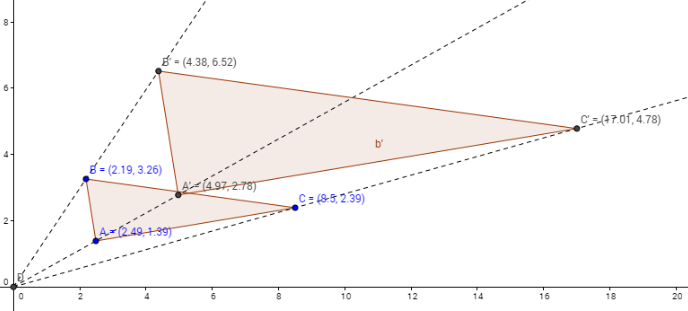• Understand that a two-dimensional figure is similar to another if the second can be obtained from the first by a sequence of rotations, reflections, translations, and dilations; given two similar two-dimensional figures, describe a sequence that exhibits the similarity between them
• How many transformations have been performed on the figure?
• How do you know which figures are congruent? Similar?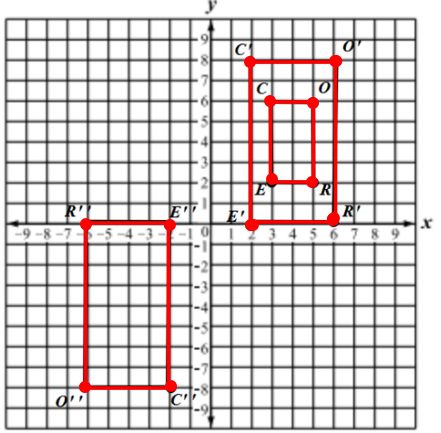• Use informal arguments to establish facts about the angle-angle criterion for similarity of triangles.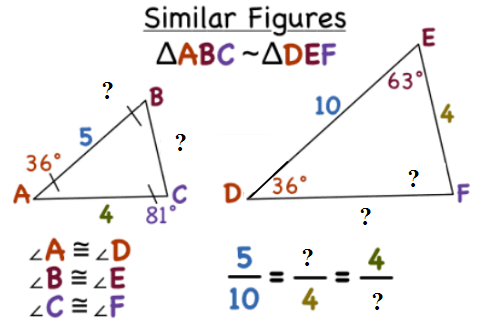### At home, your child can...

• Visit the CK12 PLIX (Play Learn Interact Xplore):

• To access the PLIX, you will need to create a free user account.
• Indirect Measurement: Treehouse Height: Use similar triangles to find the height of one object in relationship to another.

• Examine how transformations appear in dance. The online video, Grand Square, showcases two dances that were developed hundreds of years apart.
• Watch as the National Museum of Mathematics uses an image of a visitor to create a "Human Tree" using dilations. This video focuses on how similar figures can create dilations and extends student learning to explore how exponents can be used in an equation to express the proportional relationship in fractals.

*Additional Practice links support C2.0 content, but may use vocabulary or strategies not emphasized by MCPS.

## Volumes of Cones, Cylinders, and Spheres

### Students will be able to...

*Instructional videos in the hyperlinks above are meant to support C2.0 content, but may use vocabulary or strategies not emphasized by MCPS.

The Common Core State Standards require a balance of three fundamental components that result in rigorous mathematics acquisition: deep conceptual understanding, procedural skill, and mathematical applications and modeling.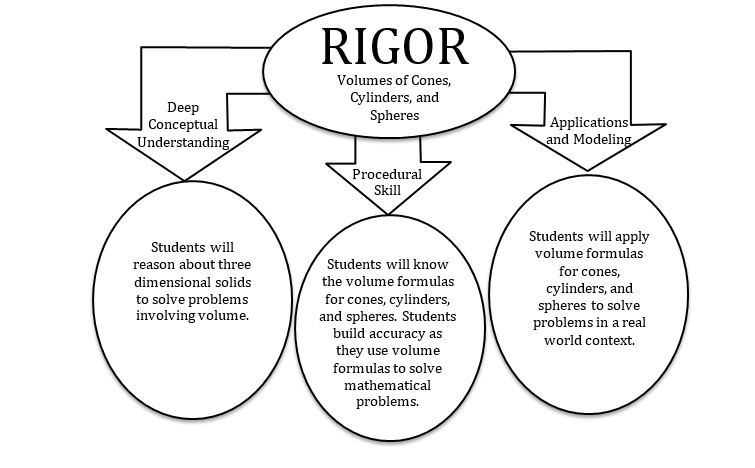### In school, your child will…

• Know the formulas for the volumes of cones, cylinders, and spheres and use them to solve real-world and mathematical problems
• Name each solid and write the volume formula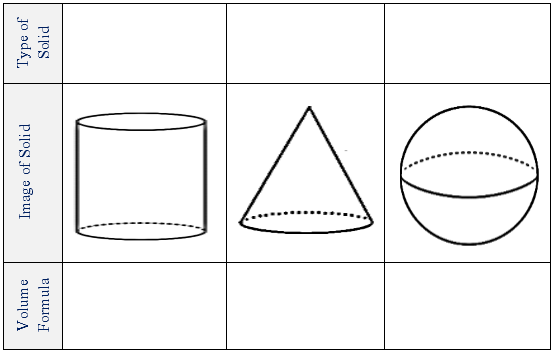•  Use the diagram below to determine which solid has the greater volume.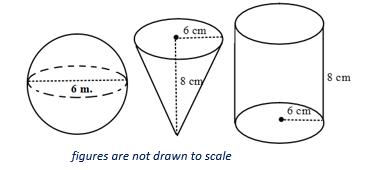• What is the ratio of the volume of the cone to the volume of the cylinder?
• What is the ratio of the volume of the sphere to the volume of the cylinder?

### At home, your child can...

• Watch the online video, Understanding Engine Size to see how the volume of cylinders is applied in the real world.
• Take a trip to the local grocery store and examine which groceries are packaged in cylinders and which are packaged in rectangular prisms. Then discuss the following questions together.
• Why do soups and pops and other food containers come in cylindrical containers?
• When food companies ship these items, do they fill the shipping boxes completely?
• When food companies ship these items, what type of package do they use? Is it a cylinder or prism?
• If we ship items in prism boxes for packaging, why do we not make containers that fit this package better? What if we shipped items in prism containers?